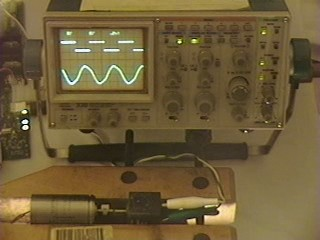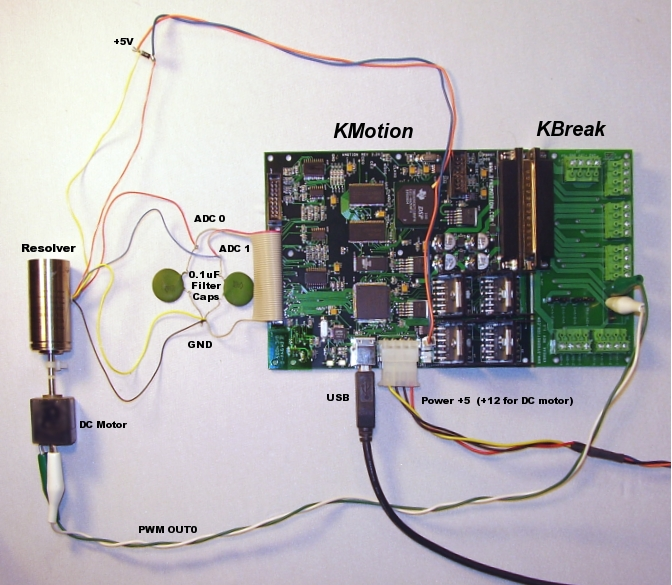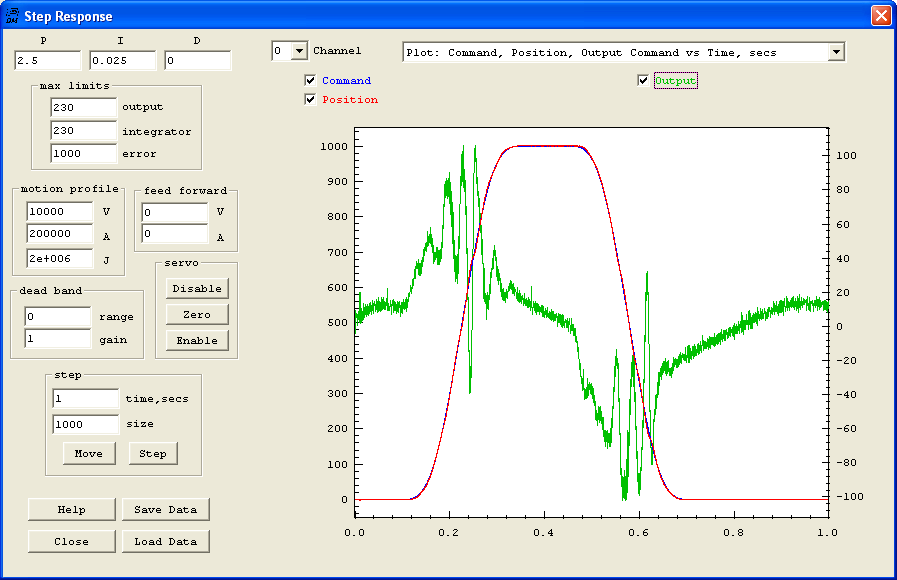# Configuring a Resolver as Input to KMotionThis example configuration shows a Resolver used as a KMotion input device. A Resolver serves a purpose much like a digital encoder. A Resolver is physically somewhat similar to a transformer with two output windings called sine and cosine outputs. For a given excitation input, the amplitude of the sine output is proportional to the sine of the mechanical rotor position, and the amplitude of the cosine output is proportional the the cosine of the mechanical rotor position. By driving the excitation coil of a Resolver and measuring the amplitude of the sine and cosine outputs, the mechanical rotor position can be determined. Although a Resolver is an analog device there are some significant advantages. A resolver is a high reliability device originally developed for military applications. Because a Resolver is able to determine the rotor position in an absolute manner for an entire shaft revolution it is not possible to "lose" position of a fraction of a shaft revolution, as with an encoder. If the position is "lost" it must be lost by a complete multiple of an entire shaft rotation. Often older CNC systems used Resolvers as their feedback device. When using KMotion to retrofit an older system with resolvers, because KMotion may be used with resolvers, it is not necessary to modify the original system to change to digital encoders.

Note: many older systems used a resolver in a somewhat reverse manner. The controller excited the sine and cosine coils in a manner such that third coil would generate a signal proportional to the difference between the desired rotor position and the actual rotor position. This difference was then used to directly drive the servo motors to reduce the error. Operating in this manner the controller has no information on the actual rotor position or the following error between the desired and actual rotor positions. A resolver originally used in this manner may still be used in the manner described in this example.

Shown below is a schematic symbol of a Resolver. The arrow indicates a varying coupling between the excitation input and the output coils as a function of rotor angle.Here is a typical resolver unit (manufactured by Singer) with 6 wires, two for each of the three coils. The wiring of a resolver can usually be determined using a simple ohmmeter. The ohm meter can be used to determine which pair of wires belong to a coil. Furthermore the sine and cosine coils should have a very similar resistance and usually different from the excitation coil. In the resolver shown below the excitation coil has a resistance of 20 ohms and the sine/cosine oils have a resistance of 37 ohms. Swapping the sine and cosine coils will simply reverse the measured motion.Resolvers may be interfaced to KMotion in one of two ways. One method is where an external circuit or module is available to excite the resolver and determine the sine and cosine magnitudes externally. In this case only the sine and cosine magnitude signals need to be connected to ADC inputs and "Resolver" input mode can be selected. For further information regarding this interface method see the Configuration Screen Setup.

A second method is where KMotion performs the excitation and synchronously samples the AC outputs to determine and track the Resolver position. The second method doesn't require an external Resolver Control Module or control circuit. Only a single diode and two filter capacitors are required. This is the method used in this example configuration. The method does require a KMotion User Program (shown below written in C) to switch the resolver excitation on and off and to sample the output waveforms at the appropriate times to determine the amplitudes.

KMotion's Aux Switch output is used in this example to excite the resolver's input coil with a 5V square wave. +5V is applied to one end of the coil and the Aux Switch drives the opposite end of the coil to ground. This applies +5V to the coil which causes current to ramp up in the coil. When the switch is turned off, current is allowed to recirculate through a diode which will cause the current to ramp down due to the coil's internal resistance. This processes is repeated every 4 User Program Time Slices (180us each) or every 720us (1.4KHz). The User Program computes the resolver position on both the positive and negative transitions so the effective update rate is 2.8 KHz. This Aux Switch output, and single diode, may be used to drive several resolvers.

The two output coils of each resolver are each connected to an ADC input with the other ends of the coils connected to ground. 0.2uF filter capacitors are used to smooth the output waveforms and should be located near the KMotion Board.

The example layout below shows the connections for a single resolver. Because KMotion has only 4 ADC inputs, KMotion is limited to interfacing to two resolvers. If more axes are required then digital encoders must be used. For testing purposes a small DC torque motor is shown connected to the resolver. The DC motor is driven from one PWM output using the +12V supply. A more likely scenario for an actual CNC retrofit would be to use the existing motor power amplifiers driven by KMotion's +/-10V DAC outputs.A wiring diagram for a complete 2 axis resolver is shown below. Also shown is the DAC output connections for connecting to external power amplifiers with +/-10 V Inputs.This is a KMotion User Program which basically loops every 4 time slices (720us), switches the 5V excitation, samples the ADC readings, computes the output magnitudes, and calls an internal function (DoResolverInput2) that computes the rotor angle and updates the axis's Position. DoResolverInput2 internally multiplies the measured angle (in Radians) by 1000/(2 pi) so that 1 shaft revolution will be seen as 1000.0 counts. This causes numeric values to have similar ranges as with digital encoders.

```	```
// Two Axis Resolver Program  1/6/08
//
// outputs square wave to both resolvers using Aux Switch Output
//
// then samples output coils near positive and negative peaks
// takes the difference to compute magnitudes
//
// these ratios are used to match the amplitudes of sine:cosine

#define RATIO0 (978.0f/768.0f)  	// size j/size k
#define RATIO1 (950.0f/709.0f)  	// size n/size m

main()
{
int i=0;
int k0,j0,k1,j1;
int m0,n0,m1,n1;

SetBit(28);  //+/-15V on
SetBitDirection(30,1);  		// configure AUX switch as output
DefineCoordSystem(0,1,-1,-1);	// Define 2 axis system

Delay_sec(0.1);					// wait for +/- 15V to be stable
for (;;)						// repeat forever
{
WaitNextTimeSlice();		// wait a few servo cycles
WaitNextTimeSlice();
Delay_sec(10e-6); 			// wait for ADC conversion to complete
SetBit(30);					// Switch the resolver excitation

//compute & track position based on measured magnitudes
DoResolverInput2(ch0,(k1-k0)*RATIO0,j1-j0);
DoResolverInput2(ch1,(m1-m0)*RATIO1,n1-n0);

WaitNextTimeSlice();		// wait a few servo cycles
WaitNextTimeSlice();
Delay_sec(10e-6);			// wait for ADC conversion to complete
ClearBit(30);				// Switch the resolver excitation

//compute & track position based on measured magnitudes
DoResolverInput2(ch0,(k1-k0)*RATIO0,j1-j0);
DoResolverInput2(ch1,(m1-m0)*RATIO1,n1-n0);

#if 0	// enable this to print the magnitudes occasionally
if (++i==1000)
{
i=0;
printf("%5.0f %5d\n",k1-k0,j1-j0);
}
#endif
}
}
```
```

The Configuration Screen input mode is shown here set to "User Input". This allows the User Program shown above to be in charge of setting the current position./

Here is a Move Plot of a move of 1000 which corresponds to 1 shaft revolution of the encoder. Not the Command (Blue) and Measured (Red) positions are nearly overlaid.Zooming in on the position gives an indication of the position resolution and noise level. The signal shown below has a range of approximately +/- 0.5 counts where 1 count is 1/1000 of a shaft resolution. So for example on a system with a 5 pitch lead screw (0.200 inches / rev) this would correspond to +/- 0.1 mils (~ 2.5um).